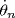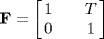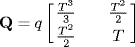# 6.4 Application of the LKF to DIFAR Buoy Bearing Estimation

The LKF can be applied to a single DIFAR buoy using its bearing observations to estimate a state vector that includes bearing and bearing rate. That is, let the state vector be defined as

(6.13)where the bearing θn is the angle between the y-axis, which points to true North, and the line drawn from an origin at the buoy to the target ship, with the convention that −180 deg <θ ≤ 180 deg.is the bearing rate of change. For this simple problem, the control variable un is not needed so the dynamic transition equation is given by

(6.14)with

(6.15)and vn−1 a zero-mean Gaussian random dynamic acceleration noise process defined by, where

(6.16)The Q used here is the dynamic noise covariance of a continuous noise process with q, the variance of the bearing acceleration noise, set at 0.1 for this example. A complete derivation of ...

Get Bayesian Estimation and Tracking: A Practical Guide now with the O’Reilly learning platform.

O’Reilly members experience live online training, plus books, videos, and digital content from nearly 200 publishers.